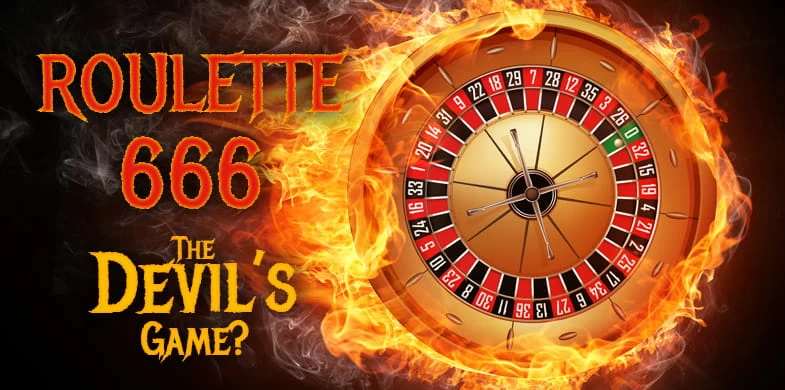# 🔥 Devil Roulette 666 🔥

#### Tomki91

##### Unique
 ️‍Roulette numbers - history of roulette 666 ️‍​However, this is not the only connection with satanic powers that is attributed to roulette. The devil's game is called primarily because of the numbers, or rather one specific number - 6, which, according to biblical rhetoric, is associated with the ruler of hell. There are several references to the satanic number in roulette. Certainly one of the most famous and associated is the fact that the sum of the numbers on the circle, ie from 0 to 36, gives the result 666. This phenomenon itself seems to be the intended action of the creators.

Still, it gets even more interesting. In fact, you can find references to a six all over the board. If we add any 3 numbers in a row (horizontally) and we continue to add hundreds, tens and ones, we always get the digit 6. Let's take an example from the second row of the board: 4 + 5 + 6 = 15; 1 + 5 = 6. The same thing happens in the last row: 34 + 35 + 36 = 105; 1 + 0 + 5 = 6. The same is true for adding diagonally spaced numbers. For example: 9 + 11 + 13 = 33; 3 + 3 = 6, or even 28 + 32 + 36 = 96; 9 + 6 = 15; 1 + 5 = 6. It's not over yet. If we apply the same addition method, adding up the numbers in each of the three dozen on the board, we also get sixes. The first dozen gives us the sum of 78, 7 + 8 = 15; 1 + 5 = 6. The numbers in the second dozen when added together give the result 222. 2 + 2 + 2 = 6. The third dozen is the sum of 366, which still gives us 3 + 6 + 6 = 15 and 1 + 5 = 6.

It's hard to believe that all these connections are accidental, and even so, it's no wonder they call roulette a devilish game. However, there are many mathematical properties of the number 666 and the number 6 that have nothing to do with religion. Anyway, the one who was the actual creator of roulette cannot be denied mental acuity, creativity and… humor.

••Koiwdcv141, meyzee and kryakrya
Super

•Tomki91
Total math sum from roulette:

0 + 1 + 2 + 3 + 4 + 5 + 6 + 7 + 8 + 9 + 10 + 11 + 12 + 13 + 14 + 15 + 16 + 17 + 18 + 19 + 20 + 21 + 22 + 23 + 24 + 25 + 26 + 27 + 28 + 29 + 30 + 31 + 32 + 33 + 34 + 35 + 36 = 666